# Convolution

## THERE IS NO PHYSICAL MEANING FOR THIS, FYI!

Just thought you woud like to know. It's used because it gives a nice way of describing the output of a linear, time-invariant system.

## Linear systems

### Requirements

homogeneity
change in input signal's amplitude results in a corresponding change in output signal's amplitude. A system is said to be homogenous if it has this property.
adding two inputs is equivalent to the corresponding outputs being added to each other. I.e.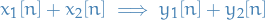For DSP, we also have:

shift invariance
shift in input implies identical shift in output

## Impulse Function Decomposition

• Input signal is decomposed into simple additive components
• System response of the input signal results in by adding the output of these components passed through the system
• A signal be decomposed as a weighted sum of basis signals
• E.g. Fourier Series

The impulse function is simply a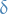function, and so we have the decomposition to be: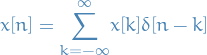1

## Matrix

For a matrix we simply do the same thing, but now consider some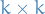matrix with all entries being zero except the middle, which is 1, to be the definition of our.

(Not sure how you do it if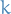is even..)

Checkout this. Scroll a bit down and you got the section about "2D Convolution".

## My view on things

1. Decompose our input signal into a set of impulses2
1. Compute output by adding the scaled and shifted impulse responses,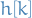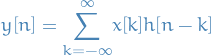3

where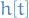is a linear time-invariant system which takes input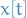.

2. If we only consider a smaller region by letting all entries forbe zero outside of this region: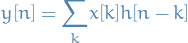4

For some finite number of. Note the differences in the summation terms.

## Why the flipping?!

The impulse response describes how each point in the input signal affects the output signal. This results in each point in the output signal being affected by points in the input signal weighted by a flipped impulse response.

That is; it's because of the maths, yo.